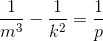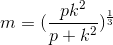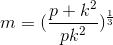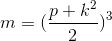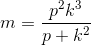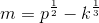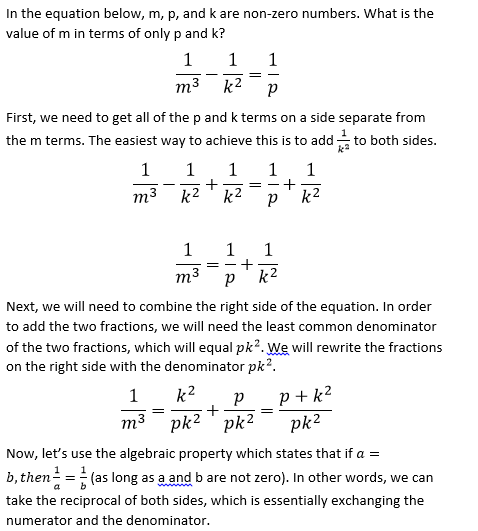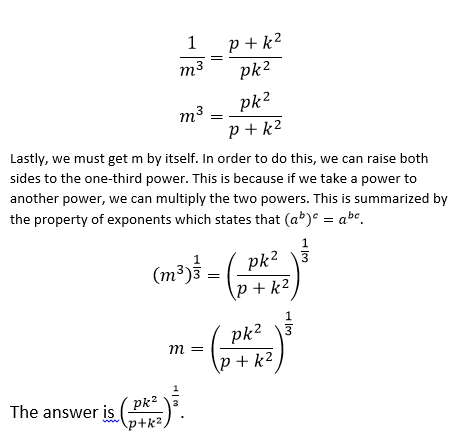## Example Questions

### Example Question #1 : How To Find The Solution To A Rational Equation With Lcd

John, Jill, and Jack are splitting a pizza. John eats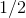of the pizza and Jill eats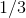of the pizza. How much of the pizza is left for Jack?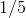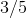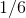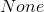Explanation:

We can write an equation for the amount of pizza eaten, withas the amount left for Jack.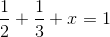To solve this equation, we must find the lowest common denominator ofand. We can list the multiples ofandto find the least common multiple: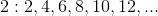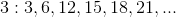We can see that the least common multiple ofandis, so we can rewrite each of the fractions with a denominator of.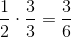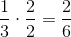When we put these fractions back into the equation, we can solve for: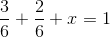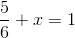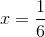### Example Question #2 : How To Find The Solution To A Rational Equation With Lcd

What is the slope of a line represented by the equation: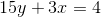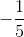Explanation:

To solve problems where you need to find the slope of a line in a given equation, change the equation so that it matches y-intercept form:For this equation, first move the 3x over to the other side of the equation.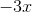The equation should now look like this: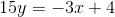Then, divide by 15 to isolate the variable.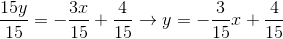Then simplify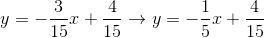Whatever number is before the x in the equation (m) is your slope.### Example Question #15 : Equations / Inequalities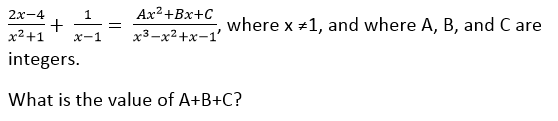–2

1

2

–1

0

2

Explanation: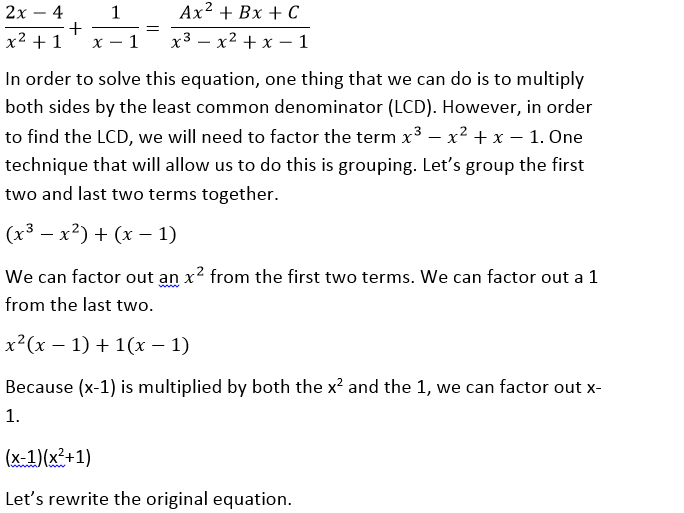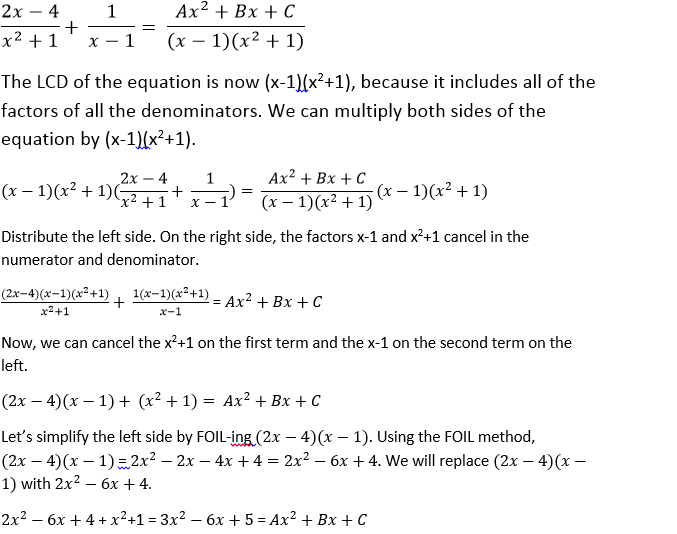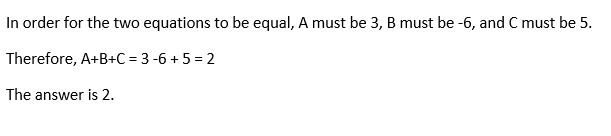### Example Question #1 : How To Find The Solution To A Rational Equation With Lcd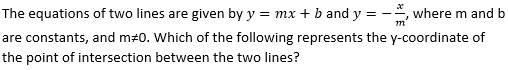–bm/(m+ 1)

–b/(+ 1)

bm/(m+ 1)

b/(m+ 1)

b/(m– 1)

b/(m+ 1)

Explanation: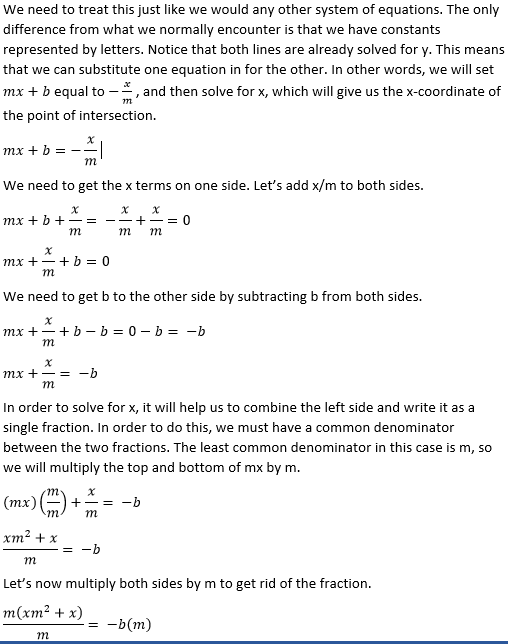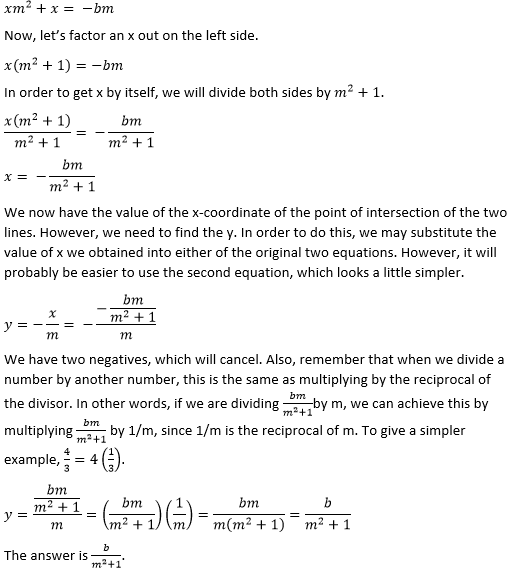### Example Question #17 : Equations / Inequalities

In the equation below,,, andare non-zero numbers. What is the value ofin terms ofand?